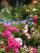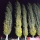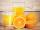Cleaning windows

Cleaning company has to wash all the windows of the school. The first day washes one-sixth of the windows of the school, the next day three more windows than the first day and the remaining 18 windows washes on the third day. Calculate how many windows have school.

Result

n =  54

Solution:

n/6+3n/6+18=n

2n = 108

n = 54

Calculated by our simple equation calculator.

Leave us a comment of example and its solution (i.e. if it is still somewhat unclear...):Be the first to comment!To solve this verbal math problem are needed these knowledge from mathematics:

Need help calculate sum, simplify or multiply fractions? Try our fraction calculator. Do you have a linear equation or system of equations and looking for its solution? Or do you have quadratic equation?

Next similar examples:

1. SeamstressThe seamstress cut the fabric into 3 parts. The first part was the eighth fabric, the second part was three-fifths of the fabric and the third part had a length of 66 cm. Calculate the original length of the fabric.
2. Unknown numberI think the number - its sixth is 3 smaller than its third.
3. RosesOn the large rosary was a third white, half red, yellow quarter and six pink. How many roses was in the rosary?
4. Vehicle tankA vehicle tank was 3/5 full of petrol. When 21 liters of fuel was added it was 5/6 full. How many liters of petrol can the tank hold?
5. PearsThere were pears in the basket, I took two-fifths of them, and left six in the basket. How many pears did I take?
6. Unknown numberIdentify unknown number which 1/5 is 40 greater than one tenth of that number.
7. FractionsThree-quarters of an unknown number are 4/5. What is 5/6 of this unknown number?
8. Equation with fractionsSolve equation: ? It is equation with fractions.
9. Equation with xSolve the following equation: 2x- (8x + 1) - (x + 2) / 5 = 9
10. EquationSolve the equation: 1/2-2/8 = 1/10; Write the result as a decimal number.
11. EqnSolve equation with fractions: 2x/3-50=40+x/4
12. UN 1If we add to an unknown number his quarter, we get 210. Identify unknown number.
13. Simple equationSolve for x: 3(x + 2) = x - 18
14. Simple equation 6Solve equation with one variable: X/2+X/3+X/4=X+4
15. TogetherGrandfather, father, and son are carpenters. They decided to make a wardrobe. Father produces it for 12 hours, his son for 15 hours, and grandfather for 10 hours. How long will they make it together?
16. PoplarHow tall is a poplar by the river, if we know that 1/5 of its total height is a trunk, 1/10th of the height is the root and 35m from the trunk to the top of the poplar?
17. TripOn the trip drank 3/10 of pupils tea, 2/5 cola, 1/4 mineral water and remaining 3 juice. How many students were on the trip?